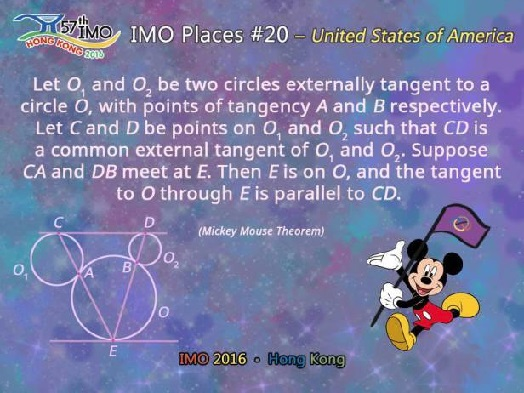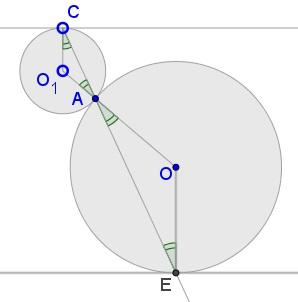# Mickey Might Be a Red Herring in the Mickey Mouse Theorem

### Source### Problem### Solution

For a proof we only need two circles, say $(O_1)\,$ and $(O).\,$ Let $CA\,$ crosses $(O)\,$ the second time at $E.\,$ Then triangles $AO_1C\,$ and $AOE\,$ are isosceles and, since $\angle CAO_1=\angle EAO,\,$ it also follows that $\angle O_1CA=\angle OEA.$Thus, $CO_1\parallel OE\,$ and, since $CO_1\,$ is perpendicular to the tangent to $(O_1)\,$ at $C,\,$ the same is true of $OE.\,$ But $OE\,$ is also perpendicular to the tangent to $(O)\,$ at $E,\,$ thus, implying that the two tangents are parallel.

We can say that $E\,$ lies on the perpendicular to the tangent at $C\,$ through $(O).\,$ Since originally, $(O_1)\,$ and $(O_2)\,$ share that tangent $CD,\,$ $DB\,$ necessarily passes through $E\,$ so that indeed $CA\,$ and $DB\,$ meet on $(O).\,$

### Remark

From the above proof it is clear that the presence of two Mickey Mouse ears in the theorem, while entertaining, is not essential. The basic result only deals with one circle $(O_1)\,$ whereas the statement remains true for any number of circles $(O_2),\,$ $(O_3),\ldots,\,$ simultaneously tangent to $(O)\,$ and to a selected tangent to $(O_1).\,$

This opens a door to consider the second ear and, thus Mickey Mouse himself, as a red herring in the eponymous theorem.

### Acknowledgment

The problem was posted by Takis Chronopoulos at the Romantics of Geometry (Οι Ρομαντικοι της Γεωμετριας) facebook group.